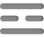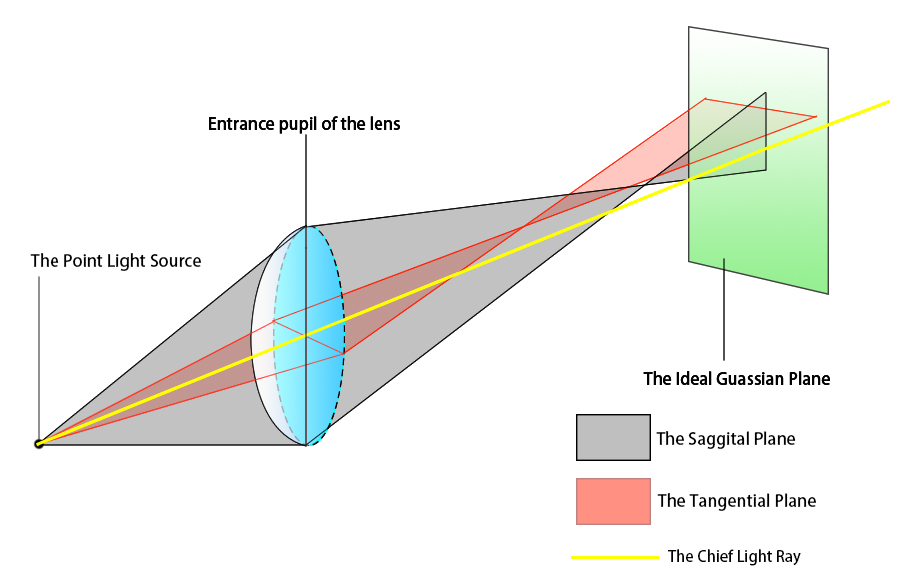# Optical Basics: Spherical Aberrations and Coma

When we are selecting or browsing online about optics, we hear the suppliers and authorized resources mentioning Optical Aberrations all the time, and there are such a fair number of them, including spherical aberrations, coma, chromatic aberration, distortion, etc. that it is prone to be confused in the maze of theories and equation. What are optical aberrations indeed?  This is a short piece of explanations about optical aberrations, and it intends to provide the most straightforward and readable detailed information on the complex and intriguing concepts of different kinds of optical aberrations. In this section, we will start with spherical aberrations and coma/comatic aberrations. Illustrative Diagrams are also injected between the paragraphs to help you understand better.

As the name suggests, optical aberration is defined as deviations from the perfect, theoretical optical model. The first thing to remember before we dive into the details is that optical aberrations are not the product of errors or inaccuracies in the manufacturing process, improper handling of the optical components, etc. but are rather inherent to the lens shapes or the properties of lights and electromagnetic waves. Even perfectly processed optical components with zero imprecision would induce optical aberration.

#### Spherical Aberration:

Spherical Aberrations result from differences in the optical paths of the light beams passing the spherical surface of an optical lens. When monochromatic light beams that are not parallel to the optical axis incident on the optical lenses, the light beams that are far from the optical axis of the optical components tend to focus at the front of the mathematical focal point of the optics (in other words, the focal points of light beams entering at the edges of the optic are closer to the light source than the theoretical focal point), and light beams that are closer to the optical axis (paraxial lights) tend to focus at the rear of the mathematical focal point of the optics. Lenses with one or more spherical sides (e.g. plano-convex lens, ball lens) are all subject to spherical aberrations. And remember to discriminate it from chromatic aberration, although both cause deviations in practical focal lengths, spherical aberrations are intrinsic for all spherical optics, even if the light source contains solely one wavelength, whilst chromatic aberrations are contributed by different refractive indexes of multi-colored lights.

The consequence of spherical aberration is the point light source becoming blurred spots on the focal plane. As we could perceive from figure 1, the magnitude of spherical aberration in lenses depends on the aperture angle. The smaller the aperture angle, the less the spherical aberration.Figure 1. Spherical Aberration

In practice, we use Numerical Aperture (NA) to indicate the magnitude of the aperture angle, the relationship could be stated as :

NA=Sinθ

Therefore, to reduce aberration in spherical lenses, the only method is to reduce the numerical aperture, however, this is often hard to realize as a reduction in NA will lead to other problems such as insufficient light throughput since a lower NA allows less light to enter the optic. A more effective approach is using an aspheric lens, which features varied radii of curvature with respect to the distance from the optical axis, to correct spherical aberration. Meniscus lenses help to attenuate spherical aberrations also. (But not as effective as aspheric optics).Figure 2. Correction of Spherical Aberration Using An Aspheric Lens

#### Coma Aberration:

Coma or Comatic Aberration is said to be present when a cone of lights emitted from a point light source is focused into a defocused, elliptical spot shaped like a comet on the focal plane. This happens when the point light source is not on the optical axis, that is, the vertex of the cone of lights is not on the axis.

As shown in the figure below, extract two planes from a cone of lights, one plane is vertical, and we name it the Tangential Plane, one plane is horizontal, and we name it the Sagittal Plane.Figure 3. Coma Aberration. The shaded area in the diagram is the Saggital Plane, while the pink area is the Tangential Plane.

Now pick the marginal light paths a and b from plane ABC, a and b are symmetrical about the chief light ray z (chief light ray is the light passing through the center of the aperture of the lens). As a and b pass through the lens, due to spherical aberration, a and b are no longer at equal perpendicular distances about z. The vertical distance between the point at which a and b intersect and the chief light ray z is defined as the sagittal coma.

Select two light paths d and f in a similar manner to the example above. d and f are at identical perpendicular distances about the chief light ray z. After passing the lens, d and f do not converge at the ideal focal plane, and the vertical distance between the point at which d and c intersect and z is the tangential coma.Figure 4. shows a detailed analysis of tangential and sagittal coma.  Plane ABC is Sagittal, and Plane BDF is Tangential. YS defined as the sagittal coma is the vertical distance between the intersection point of a and b and z, XT defined as the tangential coma, is the vertical distance between the intersection point of d and f and z.

In practice, the cross-sectional area of the light cone on the entrance pupil of the lens could be regarded as an accumulation of eccentric circles, and on the image plane these circles will form a series of overlapping circular spots of different diameters, and the centers of the circular spots are aligned on one single straight line. The resulting image on the ideal Gaussian image plane is a defocused spot shaped like a comet. The brightest vertex of the coma shape is the intersection point of paraxial narrow light beams and the chief light, and for areas apart from the chief light, the light intensity of the coma degrades.Figure 5. The formation of the Coma pattern on the image plane is when a point light source B emits light on an optical lens with a curved optical front. The final pattern appears to be a collection of circles with the outline of a cone.

Note that in most cases spherical aberration often presents simultaneous with coma. Often even if spherical aberration is eliminated, coma still exists. Decreasing the NA helps to attenuate coma. However, the best means to remove coma is using aplanatic lenses, with which perfect imaging for paraxial lights could be realized, eliminating both coma and spherical aberrations to some extent.

A more thorough understanding of comatic aberrations requires the knowledge of the Aplanatic Condition and Abbe’s Sine Condition, which are far more confusing and call for more awareness of optical fundamentals than the theories explained above. If in the future there is time sufficient, Shalom EO will post an article about the two conditions, and other optical aberrations (e.g chromatic aberrations, field curvature, distortion, etc.) in the next section, please continue to follow and support us.